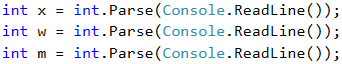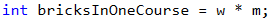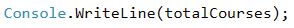Problem: Moving Bricks

The next sample exam problem is to calculate how many trolleys courses are needed to move given set of bricks (assuming the trolley has limited capacity).

Video: Moving Bricks

Watch the video lesson about solving the "Moving Bricks" problem: https://youtu.be/NuPQ0EPYjsI.

Problem Description

Construction workers have to transfer a total of x bricks. Workers are w and work simultaneously. They transport the bricks in trolleys, each with a capacity of m bricks. Write a program that reads the integers x, w, and m, and calculates what is the minimum number of courses the workers need to do to transport the bricks.

Input

On the console 3 integers are given, one per line:

• The number of bricks x is read from the first line.
• The number of workers w is read from the second line
• The capacity of the trolley m is read from the third line.

All input numbers are integers in the range [1 … 1000].

Output

Print on the console the minimum number of courses needed to transport the bricks.

Sample Input and Output

120
2
30
2 We have 2 workers, each transporting 30 bricks per course. In total, workers are transporting 60 bricks per course. To transport 120 bricks, exactly 2 courses are needed.
355
3
10
12 We have 3 workers, each transporting 10 bricks per course. In total, workers are transporting 30 bricks per course. To transport 355 bricks, exactly 12 courses are needed: 11 complete courses carry 330 bricks and the last 12th course carries the last 25 bricks.
5
12
30
1 We have 5 workers, each transporting 30 bricks per course. In total, workers are transporting 150 bricks per course. In order to transport 5 bricks, only 1 course is sufficient (although incomplete, with only 5 bricks).

The input is standard, and we only need to be careful about the sequence in which we read the data.Calculating Bricks per Course

We calculate how many bricks the workers transport in a single course:Calculating and Printing the Needed Courses

By dividing the total number of bricks transported for 1 course, we will obtain the number of courses required to carry them. We have to consider that when dividing whole numbers, the remainder is ignored and always rounded down. To avoid this, we will convert the right side of the equation to double and use the Math.Ceiling(…) function to round the result always up. When the bricks can be transferred with an exact number of courses, the division will return a whole number and there will be nothing to round. Accordingly, if not, the result of the division will be the number of exact courses but a decimal fraction. The decimal part will be rounded up and we will get the required 1 course for the remaining bricks.Finally, we print the result on the console.